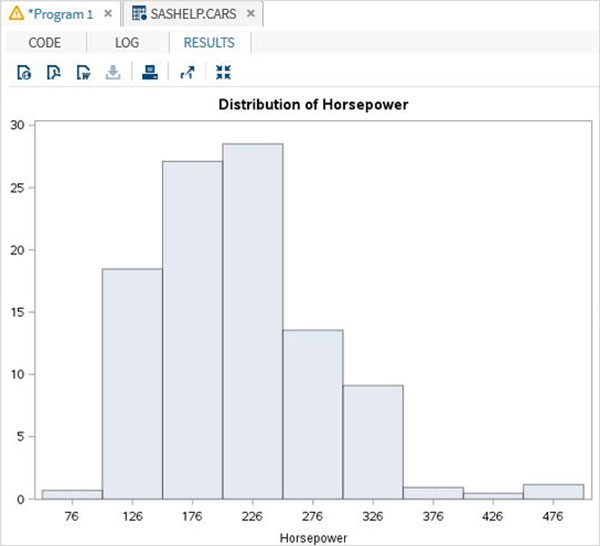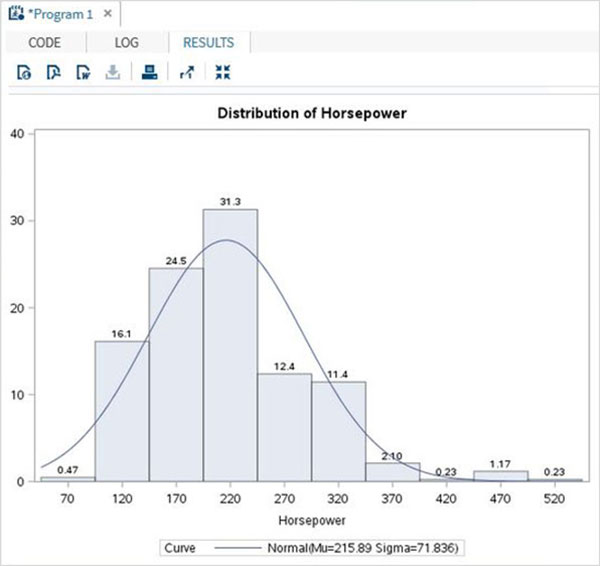# SAS - Histograms

A Histogram is graphical display of data using bars of different heights. It groups the various numbers in the data set into many ranges. It also represents the estimation of the probability of distribution of a continuous variable. In SAS the PROC UNIVARIATE is used to create histograms with the below options.

### Syntax

The basic syntax to create a histogram in SAS is −

```PROC UNIVARAITE DATA = DATASET;
HISTOGRAM variables;
RUN;
```

Following is the description of parameters used −

• DATASET is the name of the dataset used.

• variables are the values used to plot the histogram.

## Simple Histogram

A simple histogram is created by specifying the name of the variable and the range to be considered to group the values.

### Example

In the below example, we consider the minimum and maximum values of the variable horsepower and take a range of 50. So the values form a group in steps of 50.

```proc univariate data = sashelp.cars;
histogram horsepower
/ midpoints = 176 to 350 by 50;
run;
```

When we execute the above code, we get the following output −## Histogram with Curve Fitting

We can fit some distribution curves into the histogram using additional options.

### Example

In the below example we fit a distribution curve with mean and standard deviation values mentioned as EST. This option uses and estimate of the parameters.

```proc univariate data = sashelp.cars noprint;
histogram horsepower
/
normal (
mu = est
sigma = est
color = blue
w = 2.5
)
barlabel = percent
midpoints = 70 to 550 by 50;
run;
```

When we execute the above code, we get the following output −